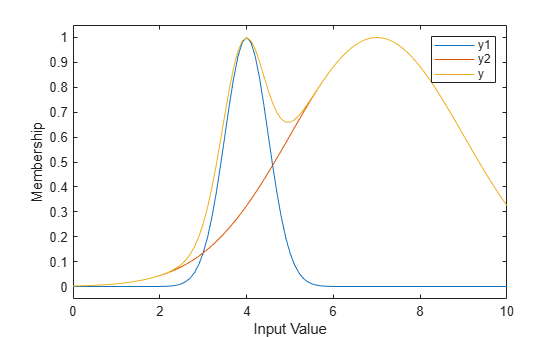# probor

Probabilistic OR

## Syntax

``y = probor(x)``

## Description

example

````y = probor(x)` returns the probabilistic OR (also known as the algebraic sum) of the columns in `x`. Within the fuzzy inference process, the `probor` function is used as either a fuzzy operator when evaluating rule antecedents or an aggregation operator when combining the output fuzzy sets from all the rules.```

## Examples

collapse all

Define the universe of discourse (input values) for the membership functions.

`x = 0:0.1:10;`

Define two Gaussian membership functions with different means and variances.

```y1 = gaussmf(x,[0.5 4]); y2 = gaussmf(x,[2 7]);```

Compute the probabilistic OR between these membership functions.

`y = probor([y1;y2]);`

Plot the results.

```plot(x,[y1;y2;y]) legend('y1','y2','y') ylim([-0.05 1.05]) ylabel('Membership') xlabel('Input Value')```## Input Arguments

collapse all

Fuzzy input values, specified as an array or a row vector.

## Output Arguments

collapse all

Probabilistic OR values, returned as a row vector with the same number of columns as `x`. Each element of `y` contains the probabilistic OR value for the corresponding column in `x`.

If `x` has one row, then `y` = `x`.

If `x = [A;B]`, where `A` and `B` are row vectors, then the `i`th element of `y` is the following algebraic sum.

`y(i) = A(i) + B(i) - A(i)*B(i);`

If `x` has more than two rows, the probabilistic OR is calculated for the first two rows. Then, the probabilistic OR is computed between the result and the next row. This process repeats for each subsequent row.

```x = [A;B;C;D] y(i) = A(i) + B(i) - A(i)*B(i); y(i) = y(i) + C(i) - y(i)*C(i); y(i) = y(i) + D(i) - y(i)*D(i);```

## Version History

Introduced before R2006a

expand all